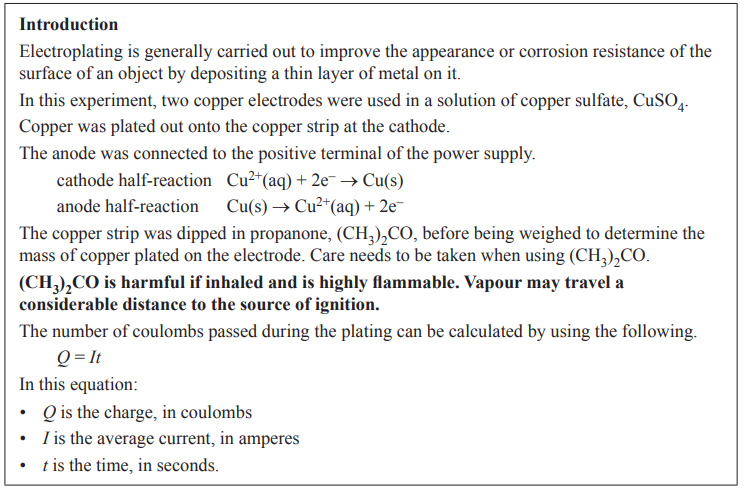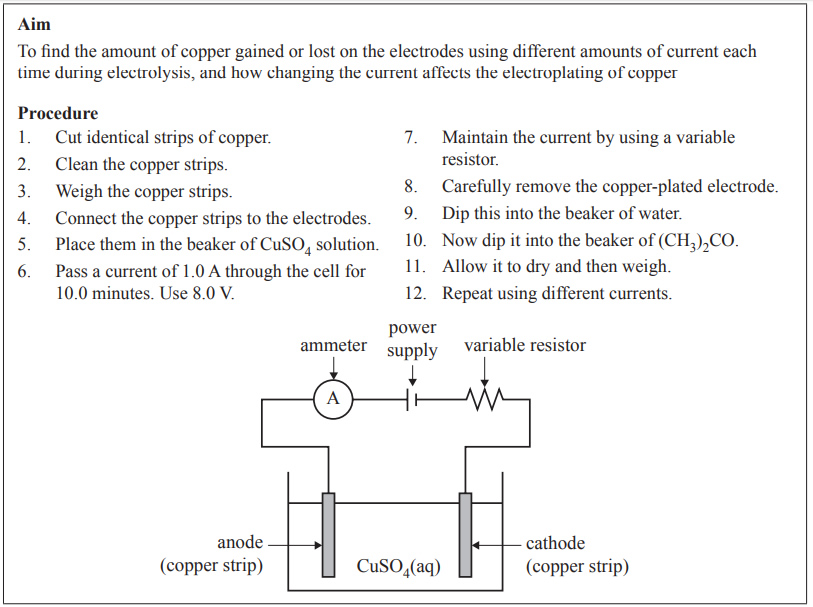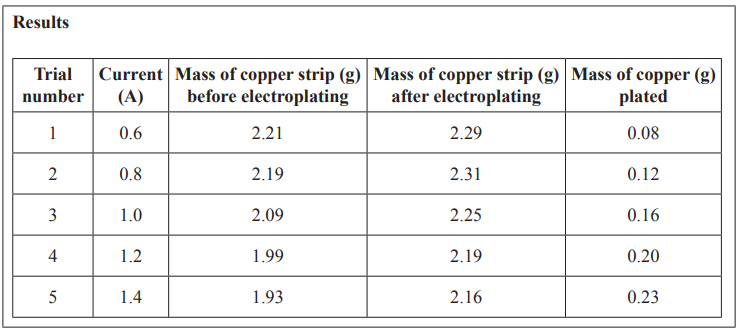Experimental technique (2018 NHT) For an extended experimental investigation, a group of students designed and carried out experiments to investigate various aspects of electroplating. Some extracts from the scientific poster produced by one of these students is shown below.a) Considering the properties of (CH3)2CO stated in the introduction, outline the safety precautions the student would take when i. using (CH3)2CO ii. disposing of (CH3)2CO .   Solution.b) i. Name the independent variable in this experiment. ii. Name a controlled variable in this experiment and state why it is important for this variable to be controlled. Solutionc) The aim and the procedure stated by the student do not match. Rewrite the aim so that it better matches the stated procedure Solutiond) i. Suggest another way of displaying the results given in the table above. ii. Describe how the class data could be used to determine the reliability of the experiment. iii. Write a suitable conclusion for the results given in the table above   Solutione) As part of a peer assessment process, a group of students reviewed the investigation and suggested a number of changes. One of these suggested changes is shown below. [Measure the mass of both the anode and the cathode before and after electroplating]. Comment on how implementing this suggested change would affect the experiment with respect to: • the validity of the experiment • the sources of error (random and systematic). Solution